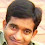## Sunday, April 9, 2017

### Interview Pearls: Interleave array in-place

I got this problem in the real interview, and I thought it was interesting. I used the divide and conquer paradigm and came up with a linearithmic solution. After the interview, I could not help thinking about potential linear solution. Read on to see what I found with a little help from the number theory.

### Problem Statement

"Given array [a1, a2, ..., an, b1, b2, ..., bn], interleave it in-place to [a1, b1, a2, b2, ..., an, bn]"
This problem is also known as "in-shuffle". Think about cutting a deck of cards into equal halves, and interleaving them perfectly, like some of poker junkies can do. It's easy to solve this problem in linear time if you can use additional memory, but the point here is to do it in-place.

### Coding practice

Try to solve this problem in linearithmic time before moving on to the solutions. I could not find a related problem on popular online judge sites (I plan on contributing it to LeetCode).

LeetCode is my most favorite destinations for algorithmic problems. It has 557 problems (at the time of this post) with test cases to automatically validate your solution correctness, as well as computational and memory complexity. Most of the problems are free, and there is a large community of programmers discussing different approaches, tips and tricks.

### 1. Brute force

We can "bubble" each element one by one, by swapping it with predecessor until it reaches the correct position. Two pointers and nested loops will do the job. The complexity is quadratic , so it won't pass Online Judge.

### 2. Divide and conquer

Try applying the above solution next time you get a deck of card to shuffle. You will learn, hands-on, how bad is quadratic with just 52 cards (and be relieved from the shuffling duty that night).

Linearithmic complexity is the next step up from quadratic, so we need to try breaking our problem into sub-problems. My interviewer sort of gave me a hint by saying that the input array size is the power of two (e.g. 16, 32, and so on). If you look at the result after interleaving, you will notice that the elements [a1, ..., an/2] and [b1, ..., bn/2] appears in the first half of the array. So, we can swap
[an/2+1, ..., an] with [b1, ..., bn/2], and then interleave the first and second halves of the array independently.
The complexity of this solution is linearithmic, so it should be good enough to pass Online Judge.

### 3. Divide and conquer - improved

The previous solution requires the array size to be power of 2. Can we improve it to work with any size? This would be a good prep work for the next solution, which provides linear shuffling time if the array size is power of 3 minus one (e.g. 8, 26, 90).

We can always split our input array into sub-arrays sized as power of 2, and apply the previous solution to each sub-array. However, we need to re-arrange elements before the split to get the correct result.
First, we find the sub-array size which is the largest power of two. We then rotate half elements of the array starting from the second half of the sub-array, until the first element in the second half of the array becomes first element in the second half of the sub-array.

Now, we are ready to apply the divide and conquer solution from the previous section.

### 4. Permutation cycles

The solution is based on this paper from Microsoft's alumni Peiyush Jain (now at Google). It interleaves an array in a set of permutation cycles, achieving linear runtime. The array size must be 3i - 1, so we'll need to shift the input array and split into sub-arrays as explained in the previous section.

We run permutation cycles for all positions m that are power of three (1, 3, 9, and so on). To determine the next element n in the cycle, we multiply m by 2n-1 modulo 3i, until we come back to the starting element. According to the number theory, that way any element is a part of only one cycle. Note that my goal here is to just show an example; dig into the paper for all that science stuff.
One wrinkle here is that, after applying permutation cycles, elements from the second half of the array appear first. We could do an extra pass to swap all pairs, however, we can get the desired result by just excluding the first and last element from the processing.
The complexity of this solution is linear, and it's as good as it ever gets.

1.2.